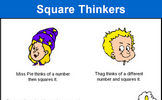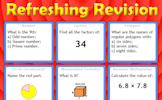# Pythagoras' Theorem

### Term 2 starting in week 11 :: Estimated time: 2 weeks

• Squares and square roots (review)
• Identify the hypotenuse of a right-angled triangle
• Determine whether a triangle is right-angled
• Calculate the hypotenuse of a right-angled triangle
• Calculate missing sides in right-angled triangles
• Use Pythagoras' theorem on coordinate axes
• Explore proofs of Pythagoras' theorem

For higher-attaining pupils:

• Use Pythagoras' theorem in 3-D shapes

This page should remember your ticks from one visit to the next for a period of time. It does this by using Local Storage so the information is saved only on the computer you are working on right now.

## Lesson Starters

Here are some suggestions for whole-class, projectable resources which can be used at the beginnings of each lesson in this block.

### 1st Lesson#### Square Thinkers

Can you find three square numbers where the third is the sum of the first two?

### 2nd Lesson#### Nine Nine Nine

Arrange the numbers 1-9 to make three 3 digit numbers that add up to 999.

### 3rd Lesson#### Red Lines

Either estimate the lengths of the red lines or, if you know how, calculate how long they are.

### 4th Lesson#### Movie Minutes

Calculations involving hours and minutes based on the runing times of popular DVDs.

### 5th Lesson#### Bizarre Triangle

By how much would the area of this triangle increase if its base was enlarged to 8cm?

### 6th Lesson#### Refreshing Revision

It is called Refreshing Revision because every time you refresh the page you get different revision questions.

Some of the Starters above are to reinforce concepts learnt, others are to introduce new ideas while others are on unrelated topics designed for retrieval practice or and opportunity to develop problem-solving skills.

White Rose ResourcesEnd of block assessments provide a quick progress check at the end of each block of learning to make sure students have understood the content covered. This Scheme of Learning was produced by White Rose Maths and is used here with permission granted on 30th June 2021.For All: